# Guide: LibreOffice Calc Part 4: Formulas

### In this fourth part of our guide on Calc, we start exploring how we can add formulas and manipulate data in a spreadsheet. This can really add magic to a spreadsheet.

Previously, we discussed how to add some visual appeal to our spreadsheets. While this can really make your spreadsheet shine, a formula can really make your spreadsheet seem magical. Formula’s don’t actually have to be all that complicated, but once you grasp the basics, you can do a lot with a spreadsheet once you throw in some math.

First, you need a general idea of what your spreadsheet is going to look like. So, I decided to try and build a simple calculator. So, I came up with the Any Two Numbers Machine. It’s by no means perfect, but we have a general idea of what we want to see.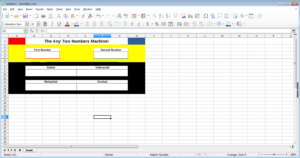The idea is that you can type in one number in one space and another number in another space. From there, the machine will add, subtract, multiply, and divide both numbers. Don’t worry, you won’t be doing any of the math. That’s the machine’s job. Of course, right now, if I put a number in both spaces, nothing will get spat out in the four blocks below. This is where the formulas come in handy.

First, we need to select the cell we want to put the resulting number in. In this case, we’re starting with the blank “Added” box.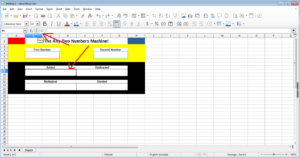Next, we are going to click on the “Sum” button which looks like a funny looking “E” (as highlighted in the screen shot). When you make that click, you’ll see the box above spit out “=Sum()”. That means it’s ready to add.

The easiest thing to do is click on the first box the user is going to insert their number. In this case, it’s cell “B4”. Next, we’re going to hold down the “CTRL” button on the keyboard and click on the other box the user is going to insert a number. In this case, it is “F4”.

Alternatively, you could also just manually type the formula “=Sum(B4,F4)” which will also point to those two cells. Either way, when you are done, hit “Enter” on your keyboard.

You should see a “0” appear in the “Added” box. This is because you are not adding any two number, so if nothing is getting added, then the answer is zero. If you click on the cell, the formula will appear on the top line while the answer will appear in the box in your spreadsheet. Go ahead and test out your machine to see if two numbers add properly.

Now, there is an alternative and even better way to add two cells. Select your addition box, then change the formula to “=B4+F4”. This will also calculate the addition of two numbers. This, of course, provides you with a great clue on how to create the formulas for the rest of the calculations.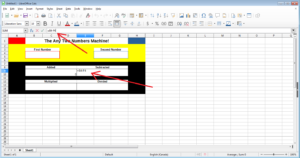Now, click on the subtraction box and insert the formula “=B4-F4”. Hit enter and you’ll have your subtraction formula covered.

For the multiply box, insert the formula “=B4*F4”. Finally, for division, use the formula “=B4/F4”.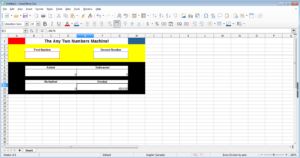You’ll likely notice that for the first three, the resulting number is zero. The division formula, however, will spit out the error message “#DIV/0!” Don’t worry about it. This is normal because you can’t mathematically divide by zero. Now, your Any Two Numbers machine should work. Just type in any two numbers and the machine will add, subtract, multiply, and divide those two numbers. Just remember to hit enter when entering the second number for the formula’s to do the work.

Pretty neat! You didn’t even have to know any math and you’ve already built a calculating machine!

Using Multiple Sheets for Formulas

Sometimes, you’ll want to move your equations off into another place in the spreadsheet. This can eliminate some of the visual clutter while producing a clean looking spreadsheet. So, let’s build a spreadsheet that tells me what I need to get a certain letter grade on a test or paper.

First, let’s create a two page spreadsheet. I’m going to designate the first sheet as the main sheet because that is what is going to be opened when you open the file. The second sheet is going to be designated the sheet for formulas.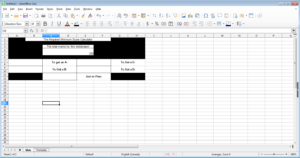First, I want to click on the cell in the “Main” that I want the result to pop out at. In this case, I’m going to start with what I need to get an A minus. First, I’m going to tell it to reference the “Formulas” sheet and tell it to look for the answer in the cell “A1”.

Important Note: Don’t use sheet names with spaces in them. If you reference the sheet with a space in its title, the space tends to mess up your formulas. So avoid sheet titles with a space in it.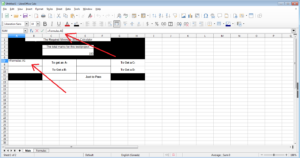So, I type in “=Formulas.A1”. Breaking this down, the cell is telling calculate to refer to the sheet “Formulas”. Next, the period tells Calc that it’s the end of the name of the sheet title. After that, the “A1” tells Calc to find the answer on the cell “A1” on that sheet. Perfect. Now, we want to put the required formula in A1 in the Formulas sheet. So, let’s click on the Formulas sheet.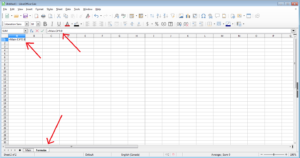Since the user is not really meant to see this sheet, we don’t have to worry as much about making this sheet pretty. Since the magic is going to happen in the A1 cell here, let’s insert the formula. Type in the formula “=Main.C4*0.9”. Breaking this down, we want Calc to look at the “Main” spreadsheet. Next, Calc is going to look at the cell “C4”. Since an A- is 90%. We want to multiply the number in C4 by 0.9. So, we insert the star and then 0.9. Now, hit enter for the formula to take effect.

You’ll notice that an answer appears here (zero). This is normal. Click on your “Main” sheet and you’ll see that the zero also appears in the proper box. This is because that cell is just peeking over at A1 on the Formulas sheet to get the answer. If you place “100” in your top box, you’ll see “90” appear in the A- part of your sheet. That definitely means that your formula works!

Now, you are free to fill in the rest of the numbers. You just need to reference different cells for your answer. In this case, I just went A1, A2, A3, A4, and A5 as I went down the grade levels. After that, in the formulas, I just used the formulas “=Main.C4*0.8”, then reduced that last integer by 0.1 as I went down the row of “A” until I reached 5. After that, who really cares about grades, right? You should just need to go back to your main sheet and test out your formula. 100 is great because the math is easy at that point.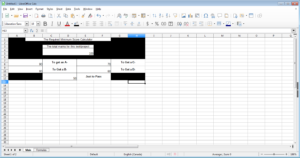For reference, if you are doing what I’ve been doing, the results should look like the above during your first test.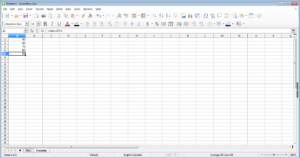Also, if you wanted to see the completed Formulas sheet. It looks like the above.

Let’s go back to something a bit more simple. What if we want to create a simple income and expense sheet? How much tot you buy vs how much you made. This is a much easier thing to accomplish in Calc.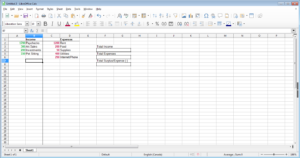First, set up the general look we want. The key here is to designate a whole column to just numbers.

Now, we want to get a total of the amount of money we make. That is actually extremely easy.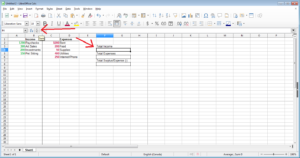First, we click on the cell we want the total to go to. Then, we click on our trusty “Sum” button.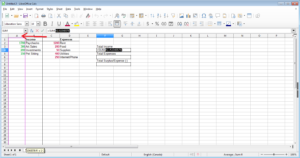From there, we just click on the “A” row. This will allow you to attach as many items as we want (well, as many items that is practical anyway). When we hit “Enter”, a total will appear in our total income box.

Next up, we’ll do the same for our expenses.

With both totals added up by Calc, we can now do a simple subtraction formula in our final box.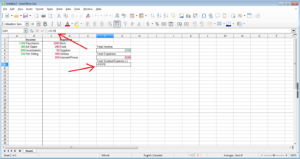When we hit enter after subtracting our two totals, we’ll see we have a surplus. If the number is minus, then we are losing money in this income and expense spreadsheet.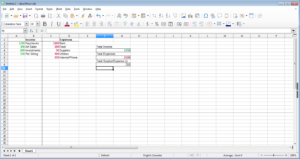The above is the result I ended up with in the Formulas sheet.

Using Formulas to Create a Visual Effect

Believe it or not, you can actually use a formula to affect the visual effect of a spreadsheet. Let’s say a spreadsheet is used as a report for an audit. This can involve a series of notes. While it is a great way to organize information, it can be visually painful to read. Take this for instance: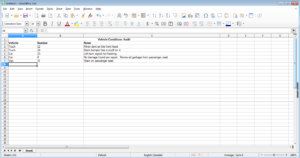If you are scanning the sheet from left to right or right to left, you can become visually lost in the information. If we were to add other columns and continue to make the sheet wider, it’ll keep getting more difficult to read. One way we can solve this is to visually break this information up a bit and make it easier to track left and right. One way we can do this is to have an alternating background colour on those rows. Unfortunately, it would be impractical to just manually insert something like that. Fortunately, it is possible to automate something like this using a formula.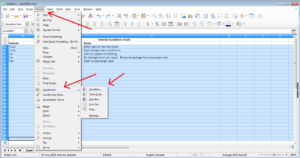First, we highlight the rows we want to adjust. Next, we click on the “Format” option in the menu bar. Next, we hover our mouse over “Conditional”. In the sub-menu, click on “Condition”.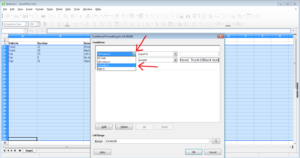In the pop-up window, click on the first drop down menu and click on “Formula Is”.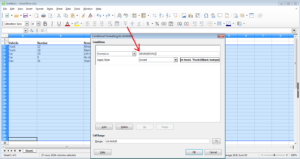In the text box right after, type in “ISEVEN(ROW())” (without quotation marks of course) like I did in the screenshot above.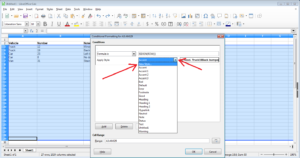In the next drop down menu next to “Apply Style”, click “New Style”.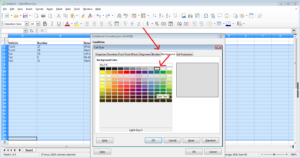In the new pop-up window, we want to click on the “Background” tab. After that, we can select a colour we want to use every other row. I’m going to stick with a light grey so that the font can still be visible regardless of which colour is used.

Now, click on the “OK” button. This closes the second pop-up window. Now, click on the “OK” button in the first pop-up window. This closes the other window.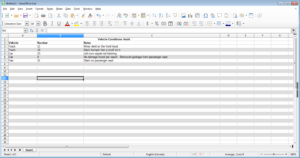Deselect the cells and you’ll see a brand new look for your report sheet! The even cooler part is the fact that if you copy the formatted rows onto the un-formatted rows, you’ll copy the pattern over cleanly and extend the alternating colour style.

We really could continue building up our formulas from there. We can figure out interest rates and even break down other kinds of mathematics. However, you get the basics with the above and even one advanced formula.

Congratulations! You now have a great idea of how to create formula’s in Calc!